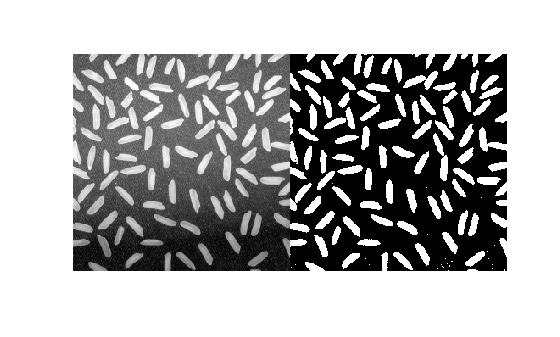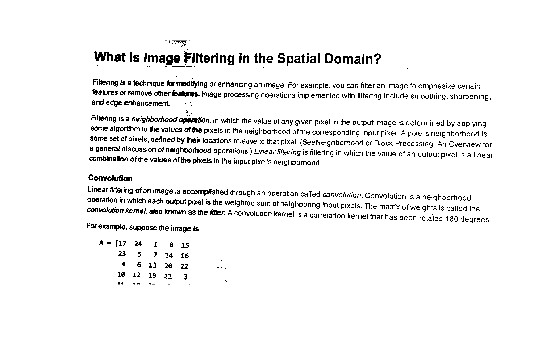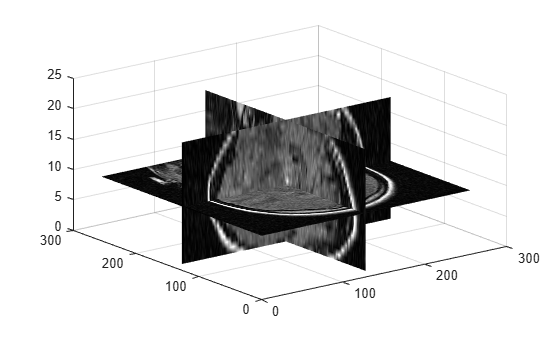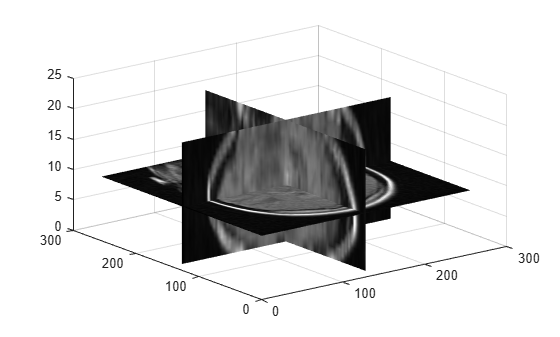## 语法

``T = adaptthresh(I)``
``T = adaptthresh(I,sensitivity)``
``T = adaptthresh(___,Name,Value)``

## 说明

``T = adaptthresh(I)` 计算二维灰度图像或三维灰度体 `I` 的局部自适应阈值。`adaptthresh` 函数基于每个像素邻域的局部均值强度（一阶统计量）选择阈值。阈值 `T` 可与 `imbinarize` 函数结合使用以将灰度图像转换为二值图像。`

``T = adaptthresh(I,sensitivity)` 使用 `sensitivity` 指定的敏感度因子计算局部自适应阈值。`sensitivity` 是范围 [0,1] 内的标量，表示通过阈值化处理将更多像素归为前景的敏感度。`

``T = adaptthresh(___,Name,Value)` 使用名称-值对组计算局部自适应阈值，以控制阈值的各个方面。`

## 示例

`I = imread('rice.png');`

`T = adaptthresh(I, 0.4);`

`BW = imbinarize(I,T);`

```figure imshowpair(I, BW, 'montage')````I = imread('printedtext.png');`

```T = adaptthresh(I,0.4,'ForegroundPolarity','dark'); figure imshow(T)``````BW = imbinarize(I,T); figure imshow(BW)``````load mristack; V = mristack;```

```figure slice(double(V),size(V,2)/2,size(V,1)/2,size(V,3)/2) colormap gray shading interp````J = adaptthresh(V,'neigh',[3 3 3],'Fore','bright');`

```figure slice(double(J),size(J,2)/2,size(J,1)/2,size(J,3)/2) colormap gray shading interp```## 输入参数

### 名称-值参数

`"bright"`

`"dark"`

`"mean"`

`"median"`

`"gaussian"`

## 输出参数

 Bradley, D., G. Roth, "Adapting Thresholding Using the Integral Image," Journal of Graphics Tools. Vol. 12, No. 2, 2007, pp.13–21.